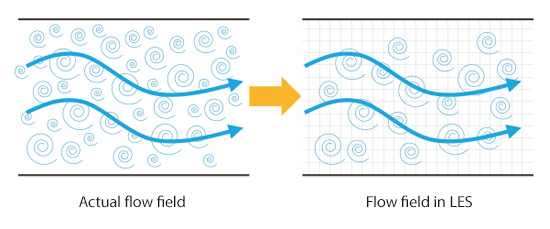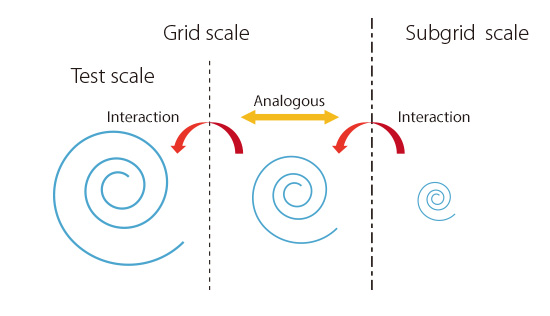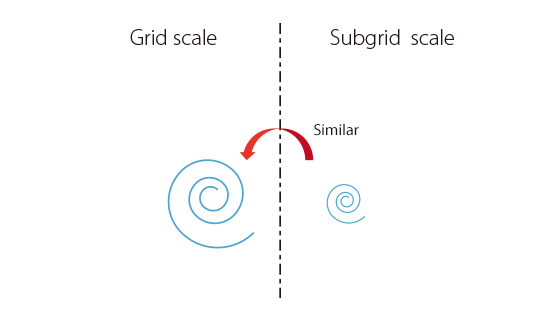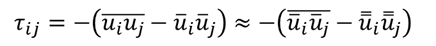# Basic Course on Turbulence and Turbulent Flow Modeling 12: 12.1 Large Eddy Simulation (LES), 12.2 Smagorinsky model, 12.3 Scale similarity model

#### 12.1: Large Eddy Simulation (LES)

We finally get to discuss Large Eddy Simulation in this column. I think, with the evolutions in computation environment, engineers in the field of product development more often perform or are eager to perform their simulations with LES. As we learned, RANS model is a method in which we make a compromise to see only the averaged flow fields and reduce computational cost. Quite often, however, we want to simulate rather transient phenomena, and RANS model has its own limit in calculation accuracy. These factors contribute to the increased expectations on LES.
In a real (turbulent) flow, many eddies of various sizes are generated. With LES, larger eddies that can be captured by mesh are calculated directly, whereas the effects of smaller eddies that cannot be captured by mesh are accounted for with modeling (Figure 12.1). The model that simulates the effects of smaller eddies is called ‘subgrid scale (SGS) model’, and a virtual stress called SGS stress is used in the modeling. Subgrid scale means the sizes smaller than mesh size. Conversely, the sizes larger than mesh size are called Grid scale (GS). Because of the direct calculations for larger eddies, LES relies less on modeling, and we can say that LES is more of a general-purpose method than RANS is.Figure 12.1: Illustration of LES

Table 12.1 sums up the differences between RANS model and LES. Since RANS model deals with time-averaged fields, transient eddies are not the subjects of calculation even if they are larger than mesh size. On the other hand, in LES, any eddies larger than mesh size are the subjects of calculation. In Chapter 8, we regarded RANS as a method to grasp roughly how many people are crossing the crosswalk. In LES, we can compass every tiny movement of each person, moment by moment, as long as we prepare mesh with the right size.

Table 12.1: Comparison between LES and RANS
 LES RANS Eddies larger than mesh size All: Direct calculation Average field: Direct calculation Varying field: Modeling Eddies smaller than mesh size Modeling Modeling

#### 12.2: Smagorinsky model

Let us learn about Smagorinsky model, which is a leading example of SGS model. Smagorinsky model uses a similar model to RANS, namely a model that considers hypothetical stress proportional to velocity gradient. That is, the stress is expressed as:

In RANS model, eddy viscosity is given by turbulence energy and turbulence dissipation rate, which are obtained by solving the separate equations from the equation for velocity. In Smagorinsky model, on the other hand, eddy viscosity is given not by solving any equation but by the following formula, assuming that it is proportional to mesh size and the magnitude of velocity gradient:

Eddy viscosity =CS ・Δ2・ (Magnitude of velocity gradient)

where, Δ is mesh size. As described in the above formula, eddy viscosity of Smagorinsky model contains a model constant CS. It is known that CS takes some value between 0.1 and 0.2 when tuned for typical flow fields; however, there is no clear guideline for determining the value, which casts uncertainty over the simulations. To resolve this inconvenience, a calculation method which adjusts CS automatically was proposed. This is the model called Dynamic Smagorinsky model (DSM). In DSM, flow fields that are calculated directly (GS) are divided into large size and small size, and CS is calculated assuming that the interaction between the two sizes is analogous to the interaction between GS and SGS (Figure 12.2). The large-size region in Grid scale is sometimes called ‘Test Scale’.Figure 12.2 Assumption in Dynamic Smagorinsky Model

#### 12.3: Scale similarity model

Smagorinsky model is a convenient model and it is still one of the leading models, but it has one big shortcoming: It cannot represent adverse energy flow from small eddies to large eddies. In a turbulent flow, larger eddies generate smaller eddies; however, in some cases, smaller eddies unite with each other to form a large eddy, which leads to adverse flow of energy --- so-called inverse cascade. In other words, the energy carried by smaller eddies becomes the energy of a large eddy, which is opposite of the usual direction of energy transfer. Smagorinsky model, however, is a model that only increases a hypothetical viscosity that is eddy viscosity; it only has the effect of taking energy away from larger eddies. Thereupon a model was proposed to enable representation of the inverse cascade: ‘Scale similarity model’. In the scale similarity model, modeling is carried out by assuming the similarity between eddies that are slightly larger than mesh size and eddies that are slightly smaller than mesh size (Figure 12.3).Figure 12.3: Scale similarity

If I write this down in a formula, it becomes:This is a model that replaces velocities in SGS stress by velocities in GS field. Since this model does not use velocity gradient or eddy viscosity like Smagorinsky model does, it can represent the inverse cascade. I suppose this model is rarely employed by commercial software, but I wanted to introduce it as one of the SGS models.
In the next column, we will discuss a calculation example of LES.